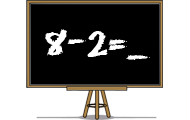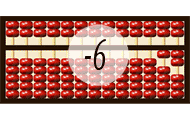# SubtractionFiltering on knowledge field: Subtraction

Math games that will teach you about mathematics, numbers and counting. Practice the basic number perception with an abacus, or practice the four rules of arithmetic. You will learn math in a joyful way by playing these games. Here we have collected all the games on the subject Math. The games suit preschool, elementary school and all the way up to high school. The games may be sorted by popularity, target group, release date or name.
0%## Minecraft math

• Multiplication
• Division
• Subtraction
• Problem solving
Count with Minecraft! You will need to multiply, divide and subtract.
0%## Subtraction

• Numbers
• Subtraction
• Mental calculation
Practice subtraction or minus in a skill training game. Find the difference between two numbers in different levels in this math game.
0%